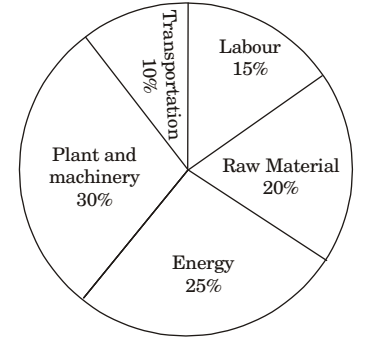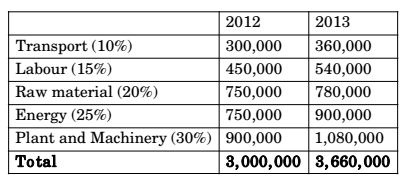## Numerical Ability

#### Numerical Ability

1. A firm producing air purifiers sold 200 units in 2012. The following pie chart presents the share of raw material, labour, energy, plant & machinery, and transportation costs in the total manufacturing cost of the firm in 2012. The expenditure on labour in 2012 is Rs. 4,50,000. In 2013, the raw material expenses increased by 30% and all other expenses increased by 20%. What is the percentage increase in total cost for the company in 2013?1.Percentage increase in total cost = 22%

##### Correct Option: APercentage increase in total cost = 22%

1. Industrial consumption of power doubled from 2000-2001 to 2010-2011. Find the annual rate of increase in percent assuming it to be uniform over the years.

1. 10 or more than 10 can't be the right answer because of compounding. Hence, 7.2 will be the right answer.

##### Correct Option: B

10 or more than 10 can't be the right answer because of compounding. Hence, 7.2 will be the right answer.

1. If 'KCLFTSB' stands for 'best of luck' and 'SHSVVDG' stands for 'good wishes', which of the following indicates 'ace the exam'?

1. KCLFTSB: BST-Best, F-Of, LCK-Luck (Reverse order)
SHSWDG: GD-Good, WSHS-Wishes (Reverse order)
Similarly “ace the Exam’- C-Ace, T-The, XM-Exam

##### Correct Option: B

KCLFTSB: BST-Best, F-Of, LCK-Luck (Reverse order)
SHSWDG: GD-Good, WSHS-Wishes (Reverse order)
Similarly “ace the Exam’- C-Ace, T-The, XM-Exam

1. Find the next term in the sequence:
13M, 17O, 19S, _______

1. In 13M - M is the 13th alphabet
17O - O is the 17th alphabet
19S - S is the 19th alphabet
Also, 13, 17, 19 are prime numbers, so next prime number will be 23 with correct answer as 23W.

##### Correct Option: C

In 13M - M is the 13th alphabet
17O - O is the 17th alphabet
19S - S is the 19th alphabet
Also, 13, 17, 19 are prime numbers, so next prime number will be 23 with correct answer as 23W.

1. In a sequence of 12 consecutive odd numbers, the sum of the first 5 numbers is 425. What is the sum of the last 5 numbers in the sequence?

1. 8th observation i s 7 × 2 = 14 more than 1st observation
9th observation is 14 more than 2nd observation
10th observation is 14 more than 3rd observation
11th observation is 14 more than 4th observation
12th observation is 14 more than 5th observation
Total 14 × 5 =70
Sum of the first five numbers = 425
Sum of last five numbers = 495

##### Correct Option: C

8th observation i s 7 × 2 = 14 more than 1st observation
9th observation is 14 more than 2nd observation
10th observation is 14 more than 3rd observation
11th observation is 14 more than 4th observation
12th observation is 14 more than 5th observation
Total 14 × 5 =70
Sum of the first five numbers = 425
Sum of last five numbers = 495